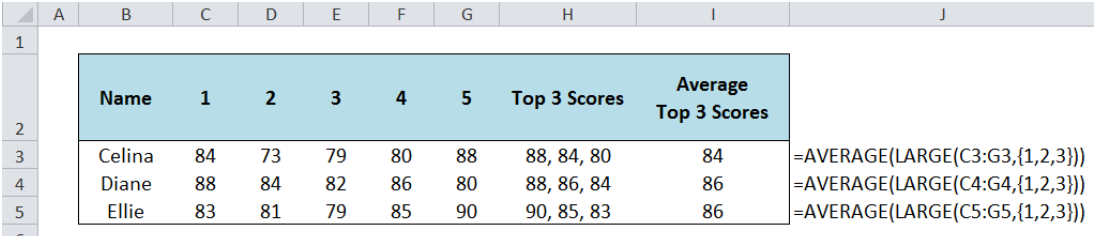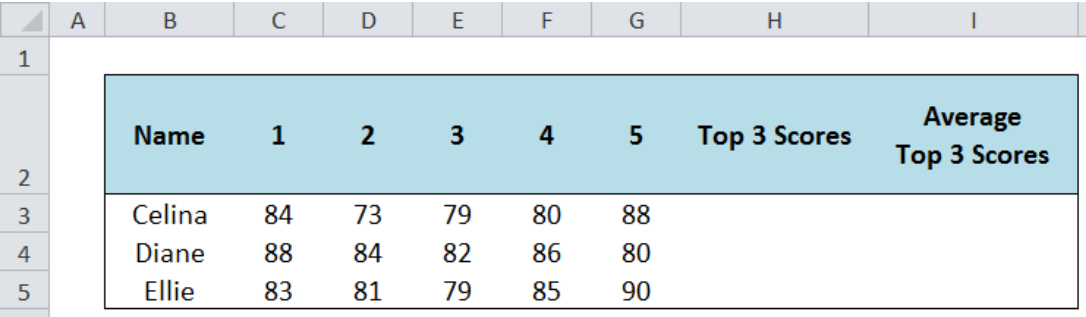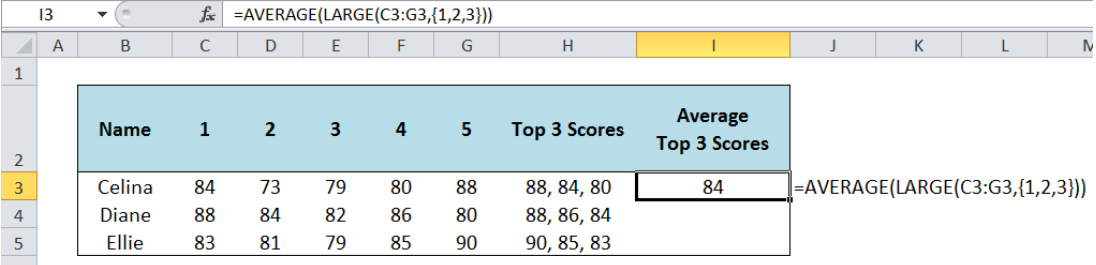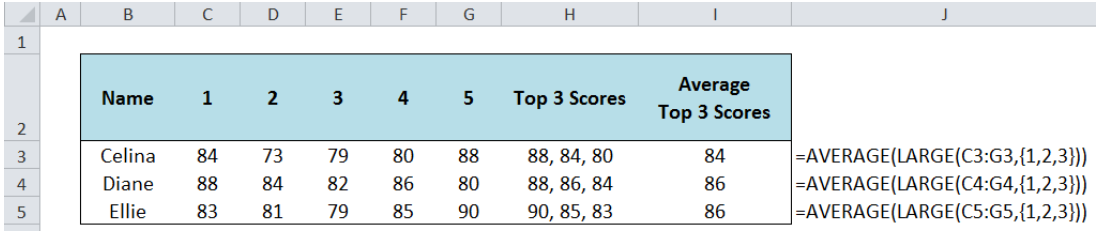Get instant live expert help with Excel or Google Sheets“My Excelchat expert helped me in less than 20 minutes, saving me what would have been 5 hours of work!”

#### Post your problem and you’ll get Expert help in seconds.

Your message must be at least 40 characters
Our professional Expert are available now. Your privacy is guaranteed.

# How to Calculate the Average Top 3 Scores in Excel

The average of the top 3 scores in any data set can be calculated by using the AVERAGE and LARGE functions in Excel. This tutorial will walk through the process.Figure 1.  Calculating the average top 3 scores in Excel

Formula: `=``AVERAGE(LARGE(C3:G3,{1,2,3}))`

## Setting up the Data

Below table shows the test scores of students in 5 sets.  We want to calculate the average top 3 scores per student.Figure 2.  Data for calculating the average top 3 scores

## Average Top 3 Scores

We will combine the two Excel functions: AVERAGE and LARGE

## AVERAGE Function in Excel

The AVERAGE function returns the average of a set of values.

### Syntax

`=AVERAGE(number1, [number2],...)`

## LARGE Function in Excel

The LARGE function returns the kth largest value in a set of data.

### Syntax

`=LARGE(array, k)`

Where array is the range of data we are evaluating to find the kth largest value

## Calculate the Average Top 3 Scores

In column H, we manually enter the top 3 scores per student so we could better understand our working formula.

In cell I3, enter the formula:

`=AVERAGE(LARGE(C3:G3,{1,2,3}))`Figure 3.  Entering the formula for the average top 3 scores

C3:G3 is the range of values we want to evaluate. We enter the array constant {1,2,3} as the value of k in the LARGE function so that the LARGE function returns an array result containing the 1st, 2nd and 3rd largest values.Figure 4.  Final result: Calculating the average top 3 scores in Excel

We can see in the example, the AVERAGE function returns the average of the three values returned by the LARGE function. The top 3 scores in row 3 are 88, 84 80 and the average is 84.

Most of the time, the problem you will need to solve will be more complex than a simple application of a formula or function. If you want to save hours of research and frustration, try our live Excelchat service! Our Excel Experts are available 24/7 to answer any Excel question you may have. We guarantee a connection within 30 seconds and a customized solution within 20 minutes.

Are you still looking for help with the Average function? View our comprehensive round-up of Average function tutorials here.

### Did this post not answer your question? Get a solution from connecting with the expert.Another blog reader asked this question today on Excelchat:
Solution examplesOn the Grade Average worksheet, select G7 and type "Average." In cell G8, use a formula to generate the average of the contents in Cells B8:F8. Use the fill handle to populate your results through G10. The syntax for the Excel Average function is the following: AVERAGE(argument1, [argument2],...). Arguments can be numbers, named ranges, cell references that contain numbers, or arrays. Select Cell A12, and enter "Class Average." Use a formula to generate the average of IP Assignment 1 in cell B12. Use the fill across handle to populate your results of averaging the other assignments to F12
Solved by Z. A. in 40 minsI need help calculating average time elapsed. It is in dd:hh:mm:ss text format and so I can't calculate using =AVERAGEIF
Solved by O. E. in 11 minsI contacted you yesterdaya bout how to use the =AVERAGE function, and for real numbers it works fine, but I tried to get the expert to explain to me how to use the =AVERAGE function when the cells have formulas in them that generate '0' value, so the AVERAGE function would ignore these cells in the averaging method, but got no answer. So, I need to know how use the =AVERAGE function so that when there are formulas in the selected cells to average, that EXCEL would ignore those cells like they have real numbers in them, which seems to work fine. Please advise...
Solved by C. J. in 30 minsI need an IF formula where, IF there is a manual adjustment in a whole column of 100% values (say someone changes one to 90%) would say "Yes" or "No". Tried to solve this with =IF(AVERAGE(...)=100%, "No", "Yes") but it is kind of sloppy).
Solved by M. H. in 20 minsIF(AVERAGE(C6+E6+G6)>=5000,TRUE,FALSE) I am using AND function and it is not giving me the correct answer
Solved by G. J. in 24 mins## Subscribe to Excelchat.coAnother blog reader asked this question today on Excelchat: